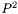#Boost C++ Libraries

...one of the most highly regarded and expertly designed C++ library projects in the world.

This is the documentation for an old version of boost. Click here for the latest Boost documentation.

## Struct template p_square_cumulative_distribution_impl

boost::accumulators::impl::p_square_cumulative_distribution_impl — Histogram calculation of the cumulative distribution with thealgorithm.

## Synopsis

```// In header: <boost/accumulators/statistics/p_square_cumulative_distribution.hpp>

template<typename Sample>
struct p_square_cumulative_distribution_impl {
// types
typedef numeric::functional::average< Sample, std::size_t >::result_type float_type;
typedef std::vector< float_type >                                        array_type;
typedef std::vector< std::pair< float_type, float_type > >               histogram_type;
typedef iterator_range< typename histogram_type::iterator >              result_type;

// construct/copy/destruct
template<typename Args> p_square_cumulative_distribution_impl(Args const &);

// public member functions
template<typename Args> void operator()(Args const &) ;
template<typename Args> result_type result(Args const &) const;
};```

## Description

A histogram of the sample cumulative distribution is computed dynamically without storing samples based on thealgorithm. The returned histogram has a specifiable amount (num_cells) equiprobable (and not equal-sized) cells.

For further details, see

R. Jain and I. Chlamtac, The P^2 algorithmus for dynamic calculation of quantiles and histograms without storing observations, Communications of the ACM, Volume 28 (October), Number 10, 1985, p. 1076-1085.

### `p_square_cumulative_distribution_impl` public construct/copy/destruct

1. ```template<typename Args>
p_square_cumulative_distribution_impl(Args const & args);```

### `p_square_cumulative_distribution_impl` public member functions

1. `template<typename Args> void operator()(Args const & args) ;`
2. `template<typename Args> result_type result(Args const & args) const;`